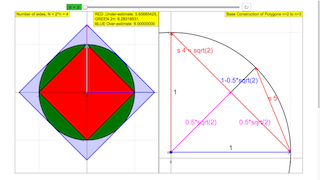Description

# Archimedes´ algorithm for the calculation of π

The simulation demonstrates the calculation of the unit circle circumference with the algorithm invented by Archimedes (287 − 221 BC). A regular polygon is inscribed to the circle, a second one is circumscribed. The circumference of the polygons can be calculated on basis of the Pythagoras theorem of rectangular triangles. The rest of the algorithm consists in drawing second roots, an art well known at that time.

The present simulation starts with squares (order n = 2, corners N = 2n = 4). A switch n+1 increases the order in steps of 1 from n = 2 to n = 12 .

The graph on the right shows the base construction of the first approximation step from square to octagon is drawn. Utilizing this drawing, it is easy to derive the formula for the inscribed polygon of the next higher order. You find the code on the page Initialization/Approximations of the EJS model to this simulation. For the 12th order (4096 corners) it is (with Math.sqrt = √ )

s = Math.sqrt(2.0-Math.sqrt(2.0+Math.sqrt(2.0+Math.sqrt(2.0

+Math.sqrt(2.0+Math.sqrt(2.0+Math.sqrt(2.0

+Math.sqrt(2.0+Math.sqrt(2.0+Math.sqrt(2.0+Math.sqrt(2.0)))))))))))

With increasing number of corners both polygons approach the circle quite quickly, and hence each other, too. Already the 32-polygons are difficult to distinguish visually.

The Reset button restores n = 2.

At the top of the windows 3 number fields show the circumference of the inscribed polygon (blue), the value (2π) of the unit circle (black), and the circumference of the circumscribed polygon (red). For n = 12 (N = 4096) they differ in the 6th decimal .

Knowing the triangle sides, it is easy to calculate the area of the triangles and as limit the area of the circle (πr2), with π for the unit circle.

Historical Importance

# Infinite series and limit value

Independent of the practical importance of a systematic calculation of π the algorithm of Archimedes is a big step forward in the basics of mathematics. It is the first documented perception of convergent infinite series and their limit value (limit).

Already in about 450 BC the presocratic philosopher Zenon of Elea theorized deeply about infinite divisibility of space and time. In this context he perplexed his contemporaries with famous Paradoxa. In the example of Achilles and the turtle the fast racer Achilles competes with a turtle that has an advance at the start. While he reaches its starting point, the turtle has again gained an advance. One can continue this reasoning ad infinitum, resulting in the conclusion that Achilles can never reach the turtle.

Interpreted in today´s mathematical understanding, the issue of the paradox is:  can a series of infinite steps, none of which is zero, lead to a finite sum (the point or the time of reaching the turtle). At the time of Zenon number theory did not include such a possibility.

Archimedes introduces the transcendental circle number as finite limit value of an infinite series, and he invents a straightforward algorithm for its calculation. The members of this series are the products of the side length of a triangle and of the number of triangles in the circle. The limit value is the product of the limit of the side length (→0) and the limit of the number of triangles (→∞).

In the case of Archimedes and the circle, the mathematical formula of the series members is rather complicated, as square root of sqare root of square root...with always the same argument − which renders it beautifully symmetric.

In Zenon´s Achilles Paradox the formula is much simpler, as the limiting value of a geometric series.

Archimedes´ algorithm includes its own proof by using 2 series that obviously converge to the same limit, an upper sum (external polygon) that is always larger than the limit and a lower sum (internal polygon) that is always smaller than the limit − for a finite order of the polygons.

The relation of the members of upper and lower sum has a simple geometric meaning, with the half angle as the decisive parameter.

Experiments

E1: Increase the order n step by step and observe the improving approximation of the circle by the polygons, and of the polygon circumference to 2π (number fields).

E2: Choose n =2 and then n = 3. Look at the construction window, and derive the formulas for the partial triangles of the square and the octagon. From this derive the formulas for the circumferences.

E3: Generalize your derivation to the n- polygon. Compare your formula with that of the Description page.

E4: Now derive the construction and the formula for the circumscribed square, octagon and n- polygon.

E5:  Reproduce Archimedes´ historical way by starting with a triangle instead of a square.

E6: Derive formulas for the approximative calculation of the area of the partial triangles, and hence of the area of the circle.

Authors

This file was created by Dieter Roess in February 2010.

This simulation is part of

– Plus 2000 Examples from Physics”

ISBN 978-3-11-025005-3, Walter de Gruyter GmbH & Co. KG

### Translations

Code Language Translator Run### Software Requirements

SoftwareRequirements

 Android iOS Windows MacOS with best with Chrome Chrome Chrome Chrome support full-screen? Yes. Chrome/Opera No. Firefox/ Samsung Internet Not yet Yes Yes cannot work on some mobile browser that don't understand JavaScript such as..... cannot work on Internet Explorer 9 and below

### CreditsDieter Roess - WEH- Foundation; Tan Wei Chiong; Loo Kang Wee

[text]

### For Teachers

This simulation allows you to change the number n with a slider, where the regular polygon used to approximate 2π has 2^n sides.

The graph on the left shows the graphical approximation, and the top right corner shows the lower bound, upper bound, and the actual value of 2π (correct to 9 digits).

The graph on the right shows how the polygon is constructed from n = 2 to n = 3 using Pythagoras' Theorem.

Research

[text]

[text]

### Version:

1. http://weelookang.blogspot.sg/2016/02/vector-addition-b-c-model-with.html improved version with joseph chua's inputs
2. http://weelookang.blogspot.sg/2014/10/vector-addition-model.html original simulation by lookang

[text]

### end faq